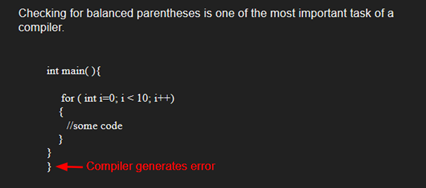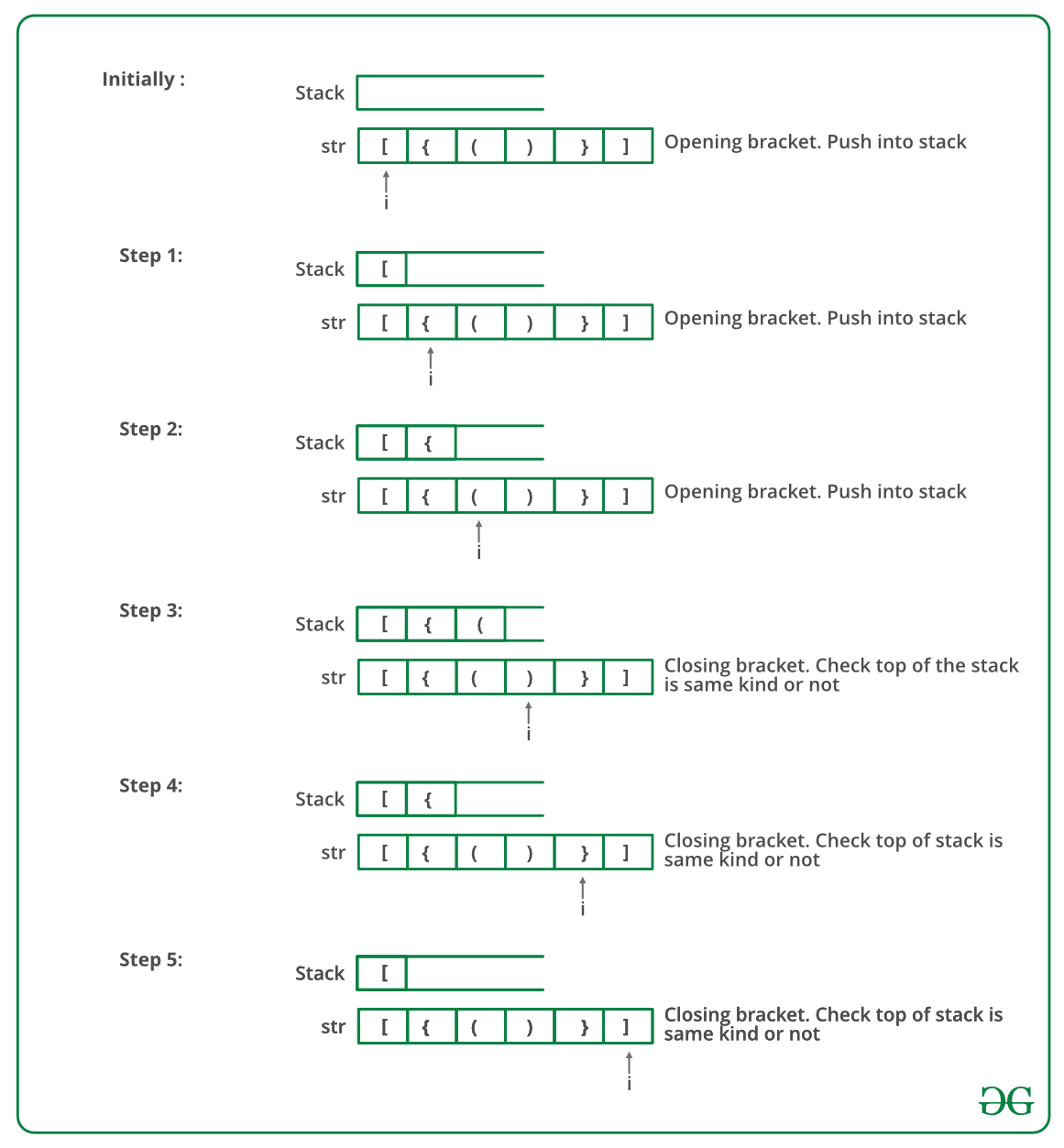Open in App
Not now

# C# Program To Check For Balanced Brackets In An Expression (Well-Formedness) Using Stack

• Last Updated : 15 Dec, 2021

Given an expression string exp, write a program to examine whether the pairs and the orders of “{“, “}”, “(“, “)”, “[“, “]” are correct in exp.

Example

Input: exp = “[()]{}{[()()]()}”
Output: Balanced

Input: exp = “[(])”
Output: Not BalancedAlgorithm:

• Declare a character stack S.
• Now traverse the expression string exp.
1. If the current character is a starting bracket (‘(‘ or ‘{‘ or ‘[‘) then push it to stack.
2. If the current character is a closing bracket (‘)’ or ‘}’ or ‘]’) then pop from stack and if the popped character is the matching starting bracket then fine else brackets are not balanced.
• After complete traversal, if there is some starting bracket left in stack then “not balanced”

Below image is a dry run of the above approach:Below is the implementation of the above approach:

## C#

 `// C# program for checking ` `// balanced Brackets ` `using` `System; ` `using` `System.Collections.Generic; ` ` `  `public` `class` `BalancedBrackets { ` `    ``public` `class` `stack { ` `        ``public` `int` `top = -1; ` `        ``public` `char``[] items = ``new` `char``; ` ` `  `        ``public` `void` `push(``char` `x) ` `        ``{ ` `            ``if` `(top == 99)  ` `            ``{ ` `                ``Console.WriteLine(``"Stack full"``); ` `            ``} ` `            ``else` `{ ` `                ``items[++top] = x; ` `            ``} ` `        ``} ` ` `  `        ``char` `pop() ` `        ``{ ` `            ``if` `(top == -1)  ` `            ``{ ` `                ``Console.WriteLine(``"Underflow error"``); ` `                ``return` `'�'``; ` `            ``} ` `            ``else`  `            ``{ ` `                ``char` `element = items[top]; ` `                ``top--; ` `                ``return` `element; ` `            ``} ` `        ``} ` ` `  `        ``Boolean isEmpty() ` `        ``{ ` `            ``return` `(top == -1) ? ``true` `: ``false``; ` `        ``} ` `    ``} ` ` `  `    ``// Returns true if character1 and character2 ` `    ``// are matching left and right brackets */ ` `    ``static` `Boolean isMatchingPair(``char` `character1, ` `                                  ``char` `character2) ` `    ``{ ` `        ``if` `(character1 == ``'('` `&& character2 == ``')'``) ` `            ``return` `true``; ` `        ``else` `if` `(character1 == ``'{'` `&& character2 == ``'}'``) ` `            ``return` `true``; ` `        ``else` `if` `(character1 == ``'['` `&& character2 == ``']'``) ` `            ``return` `true``; ` `        ``else` `            ``return` `false``; ` `    ``} ` ` `  `    ``// Return true if expression has balanced ` `    ``// Brackets ` `    ``static` `Boolean areBracketsBalanced(``char``[] exp) ` `    ``{ ` `        ``// Declare an empty character stack */ ` `        ``Stack<``char``> st = ``new` `Stack<``char``>(); ` ` `  `        ``// Traverse the given expression to ` `        ``//   check matching brackets ` `        ``for` `(``int` `i = 0; i < exp.Length; i++)  ` `        ``{ ` `            ``// If the exp[i] is a starting ` `            ``// bracket then push it ` `            ``if` `(exp[i] == ``'{'` `|| exp[i] == ``'('` `                ``|| exp[i] == ``'['``) ` `                ``st.Push(exp[i]); ` ` `  `            ``//  If exp[i] is an ending bracket ` `            ``//  then pop from stack and check if the ` `            ``//   popped bracket is a matching pair ` `            ``if` `(exp[i] == ``'}'` `|| exp[i] == ``')'` `                ``|| exp[i] == ``']'``) { ` ` `  `                ``// If we see an ending bracket without ` `                ``//   a pair then return false ` `                ``if` `(st.Count == 0)  ` `                ``{ ` `                    ``return` `false``; ` `                ``} ` ` `  `                ``// Pop the top element from stack, if ` `                ``// it is not a pair brackets of ` `                ``// character then there is a mismatch. This ` `                ``// happens for expressions like {(}) ` `                ``else` `if` `(!isMatchingPair(st.Pop(), ` `                                         ``exp[i])) { ` `                    ``return` `false``; ` `                ``} ` `            ``} ` `        ``} ` ` `  `        ``// If there is something left in expression ` `        ``// then there is a starting bracket without ` `        ``// a closing bracket ` ` `  `        ``if` `(st.Count == 0) ` `            ``return` `true``; ``// balanced ` `        ``else`  `        ``{  ` `            ``// not balanced ` `            ``return` `false``; ` `        ``} ` `    ``} ` ` `  `    ``// Driver code ` `    ``public` `static` `void` `Main(String[] args) ` `    ``{ ` `        ``char``[] exp = { ``'{'``, ``'('``, ``')'``, ``'}'``, ``'['``, ``']'` `}; ` ` `  `        ``// Function call ` `        ``if` `(areBracketsBalanced(exp)) ` `            ``Console.WriteLine(``"Balanced "``); ` `        ``else` `            ``Console.WriteLine(``"Not Balanced "``); ` `    ``} ` `} ` ` `  `// This code is contributed by 29AjayKumar`

Output

`Balanced`

Time Complexity: O(n)
Auxiliary Space: O(n) for stack.

Please refer complete article on Check for Balanced Brackets in an expression (well-formedness) using Stack for more details!

My Personal Notes arrow_drop_up
Related Articles